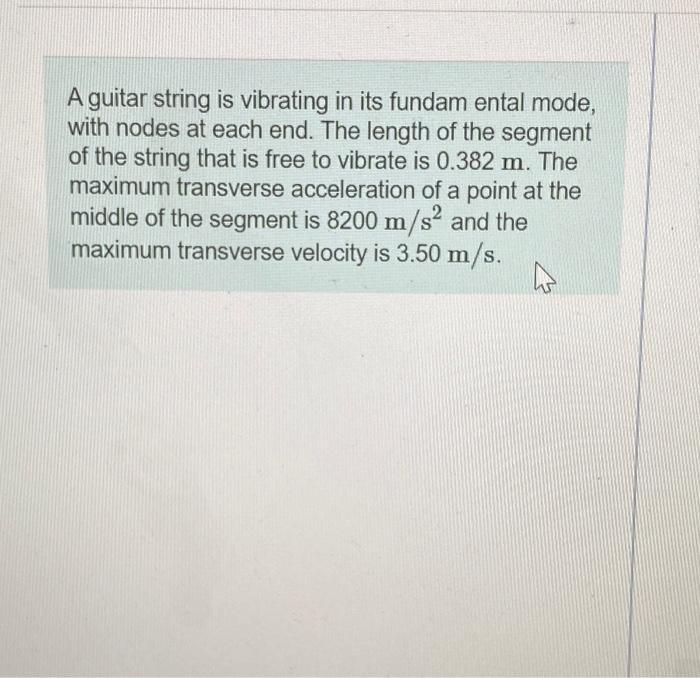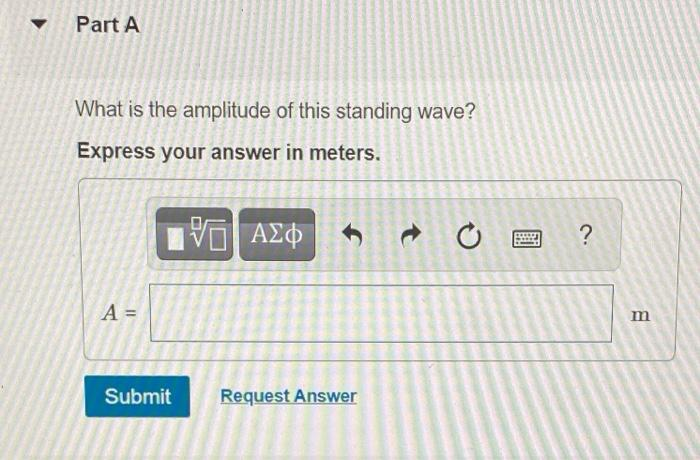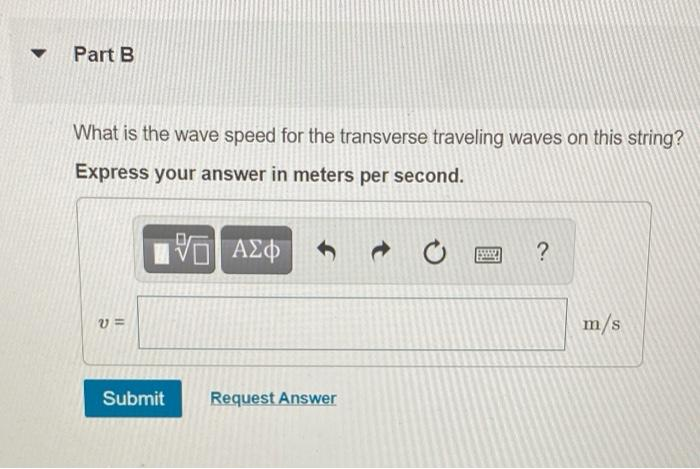# Question Solved1 Answerplease answer correctly A guitar string is vibrating in its fundam ental mode, with nodes at each end. The length of the segment of the string that is free to vibrate is 0.382 m. The maximum transverse acceleration of a point at the middle of the segment is 8200 m/s2 and the maximum transverse velocity is 3.50 m/s. w Part A What is the amplitude of this standing wave? Express your answer in meters. 10 ΑΣΦ o ? A = m Submit Request Answer Part B What is the wave speed for the transverse traveling waves on this string? Express your answer in meters per second. VOA t ? v= m/s Submit Request AnswerTranscribed Image Text: A guitar string is vibrating in its fundam ental mode, with nodes at each end. The length of the segment of the string that is free to vibrate is 0.382 m. The maximum transverse acceleration of a point at the middle of the segment is 8200 m/s2 and the maximum transverse velocity is 3.50 m/s. w Part A What is the amplitude of this standing wave? Express your answer in meters. 10 ΑΣΦ o ? A = m Submit Request Answer Part B What is the wave speed for the transverse traveling waves on this string? Express your answer in meters per second. VOA t ? v= m/s Submit Request Answer
More
Transcribed Image Text: A guitar string is vibrating in its fundam ental mode, with nodes at each end. The length of the segment of the string that is free to vibrate is 0.382 m. The maximum transverse acceleration of a point at the middle of the segment is 8200 m/s2 and the maximum transverse velocity is 3.50 m/s. w Part A What is the amplitude of this standing wave? Express your answer in meters. 10 ΑΣΦ o ? A = m Submit Request Answer Part B What is the wave speed for the transverse traveling waves on this string? Express your answer in meters per second. VOA t ? v= m/s Submit Request Answer